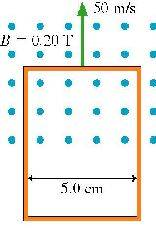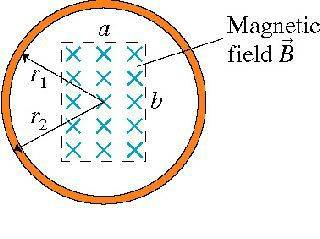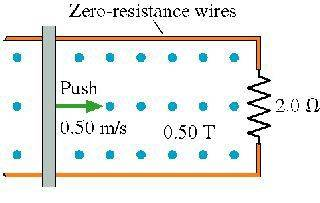# Some magnetic field/induction questions

## Homework Statement

Problem 33.12
The loop in the figure is being pushed into the 0.20 T magnetic field at 50 m/s. The resistance of the loop is 0.1$$\Omega$$.

What is the magnitude (and direction) of the current in the loop?Problem 33.24
What is the magnetic flux through the loop shown in the figureProblem 33.28

A 100-turn, 2.0-cm-diameter coil is at rest in a horizontal plane. A uniform magnetic field away from vertical increases from 0.50 T to 1.50 T in 0.60 s. What is the induced emf (in mV) in the coil?

Problem 33.44A) How big is the pushing force?
B) How much power does the pushing force supply to the wire?
C) What is the magnitude of the induced current?
D) What is the direction of the induced current?
E) How much power is dissipated in the resistor?

## Homework Equations

$$I = \frac{vLB}{R}$$

$$\Phi_m = ABcos\vartheta$$

$$E = \frac{d \Phi}{dt}$$

$$F_{push} = \frac{v L^2 B^2}{R}$$

$$P_{input} = F_push*v = \frac{v^2 L^2 B^2}{R}$$

## The Attempt at a Solution

Problem 33.12

$$I = \frac{vLB}{R} = = \frac{(50)(0.05)(0.2)}{0.1} = 5A$$ and the direction is clock wise due to the right hand rule

Problem 33.24

$$A = \pi r^2$$

$$\Phi_m = ABcos\vartheta = \pi r^2 B cos \vartheta$$ probably wrong

Problem 33.28

$$E = \frac{d \Phi}{dt} = \frac{d (ABcos\vartheta)}{dt} = - \pi r^2 \frac{dB}{dt}sin\vartheta$$

$$\frac{dB}{dt} = \frac{1}{.6} = 1.67 T$$

I can "ignore" the negative sign because I just need the absolute value, and the derivative of cos = -sin

*I forgot the number ot turns in the previous equation, but I added them in the next one*

$$N \pi r^2 \frac{dB}{dt}sin\vartheta = (100) \pi 0.1^2 (1.67) sin60 = 45mV$$

Problem 33.44

A) $$F_{push} = \frac{v L^2 B^2}{R} = \frac{(0.5) (0.1)^2 (0.5)^2}{2} = 6.25 x 10^-4 N$$

B) $$P_{input} = F_{push}*v = \frac{v^2 L^2 B^2}{R} = \frac{(0.5)^2 (0.1)^2 (0.5)^2}{2} = 3.125 x 10^4 W$$

C) $$I = \frac{vLB}{R} = \frac{(0.5)((0.1)(0.5)}{2} = 1.25 x 10^2 A$$

D) Using the right hand rule, the direction would be clockwise

E) $$P_dissipated = P_{input} = 3.125 x 10^4 W$$

Last edited:

Chi Meson
Homework Helper
12 is correct but your reasoning for the direction of current is too simple, and yourincorrect answer for the direction of current in 44 reveals that you were just lucky in 12.

To determine the direction of the current you have to figure the direction of the change in flux. In 12 the flux is out of the page and increasing. That means the change in flux is out of the page. In 44, the flux is out of the page and decreasing (fewer lines within the loop) which means the change in flux is IN to the page. The induced current produces a magnetic field that opposes the change in flux (Lentz' Law). So in 12, the current produces a B into the page and is therefore clockwise. In 44 the current produces a B out of the page, counterclockwise.

And 24: Very simple. THink of flux as the quantification of the magnetic field lines within a loop. Flux=BA. If the magnetic field is confined within the rectangle ab, then the area in which the field exists is simple. THe radius of the loop does not matter here, since the B-field is contained within the smaller area.

Last edited:
To determine the direction of the current you have to figure the direction of the change in flux. In 12 the flux is out of the page and increasing. That means the change in flux is out of the page. In 44, the flux is out of the page and decreasing (fewer lines within the loop) which means the change in flux is IN to the page. The induced current produces a magnetic field that opposes the change in flux (Lentz' Law). So in 12, the current produces a B into the page and is therefore clockwise. In 44 the current produces a B out of the page, counterclockwise.

how do you know its decreasing? Is it because the closed loop becomes smaller as the wire is moving to the right?

and would the magnetic flux be (in 24):

$$\Phi_m = AB = (ab)B ?$$

Chi Meson
Homework Helper
how do you know its decreasing? Is it because the closed loop becomes smaller as the wire is moving to the right?
In this case, yes. The change in flux can be viewed as a change in the number of field lines within the loop. Either the loop decreases in size, or it moves out of the field, or the field gets weaker (in doing so, the field lines get less dense), or the loop rotates; in each case the number of lines through the loop decreases, and the change in flux is opposite to the direction of the field.

and would the magnetic flux be (in 24):

$$\Phi_m = AB = (ab)B ?$$
That's how I see it.

In this case, yes. The change in flux can be viewed as a change in the number of field lines within the loop. Either the loop decreases in size, or it moves out of the field, or the field gets weaker (in doing so, the field lines get less dense), or the loop rotates; in each case the number of lines through the loop decreases, and the change in flux is opposite to the direction of the field.

I see what you are saying. So if it's out of the page, would it be clock wise and if it's into the page, would it always be counter clock - wise?

and how do the reasoning/answers are for the other 2 questions (if it's not too much trouble)?

any help for the last 2 questions?

dynamicsolo
Homework Helper
Problem 33.28

A 100-turn, 2.0-cm-diameter coil is at rest in a horizontal plane. A uniform magnetic field away from vertical increases from 0.50 T to 1.50 T in 0.60 s. What is the induced emf (in mV) in the coil?
...

$$N \pi r^2 \frac{dB}{dt}sin\vartheta = (100) \pi 0.1^2 (1.67) sin60 = 45mV$$

Your correction to the "coil" version of Faraday's Law is fine. But where does the "sin 60" term come from? Is there a phrase missing from the problem statement?

dynamicsolo
Homework Helper
Problem 33.44

A) $$F_{push} = \frac{v L^2 B^2}{R} = \frac{(0.5) (0.1)^2 (0.5)^2}{2} = 6.25 x 10^-4 N$$

I suspect there was some more text that went with this diagram, saying something to the effect that the bar is moving at a constant speed. This means that the net force on the bar is zero, and thus the applied force exactly balances the magnetic force acting on the induced current through the bar. (This is a phenomenon sometimes referred to as "magnetic drag".) This is what permits you to write the equation you have shown.

It seems to be absent from the diagram, but I take it that the sliding bar has zero resistance and a width of 10 cm. = 0.1 m. If this is so, your result is correct.

B) $$P_{input} = F_{push}*v = \frac{v^2 L^2 B^2}{R} = \frac{(0.5)^2 (0.1)^2 (0.5)^2}{2} = 3.125 x 10^4 W$$

Since P = F · v , and the directions of the applied force and the bar's velocity are parallel, then you can simply write P = Fv . You've just worked out F, which is both the magnitude of the applied force and the magnetic force on the induced current through the bar, so you could just take your result from part A and multiply it by v to get

P = (6.25·10^-4 N)·(0.5 m/sec) = 3.13·10^-4 J/sec or W (0.31 mW).

C) $$I = \frac{vLB}{R} = \frac{(0.5)((0.1)(0.5)}{2} = 1.25 x 10^2 A$$

The equation is correct (and, in fact, you'd have worked out this part before part A), but you've dropped a minus sign in your power of ten...

D) Using the right hand rule, the direction would be clockwise

I see you've already hashed this issue out with Chi Meson...

E) $$P_{dissipated} = P_{input} = 3.125 x 10^4 W$$

You have also dropped the minus sign in the power of ten here... (EDIT: As I look again, I see you did in Part B also.)

Ah, this statement is true, but you must demonstrate that it is! What is the current through the resistance in this loop? How much power will that resistance dissipate?

Last edited:
I already worked out 33.28. I forgot to post saying it's going at 60 degrees, and I also didnt need to find its derivative sense cos60 is a constant.

and yea, I forgot to include the desciption text in 33.44:

The 10-cm-wide, zero-resistance slide wire shown in the figure is pushed toward the 2 $$\Omega$$resistor at a steady speed of 0.50 m/s. The magnetic field strength is 0.50 T.

Last edited:
dynamicsolo
Homework Helper
I already worked out 33.28. I forgot to post saying it's going at 60 degrees, and I also didnt need to find its derivative sense cos60 is a constant.

So it is 60 degrees off the vertical then. OK, and yes, the only term to be differentiated is dB/dt.

and yea, I forgot to include the desciption text in 33.44:

The 10-cm-wide, zero-resistance slide wire shown in the figure is pushed toward the 2 $$\Omega$$resistor at a steady speed of 0.50 m/s. The magnetic field strength is 0.50 T.

Don't forget part E. It's true the power dissipated by the resistor must equal the power of the applied force, since the bar is only moving at constant speed (thus no change in kinetic energy). But this part of the problem is asking you to evaluate the effect of ohmic (resistive) heating in the 2-ohm resistor...

(I'm buggin' you on this because, if this were an exam, you'd get little credit for simply declaring that P_diss = P_input.)

Last edited:
(I'm buggin' you on this because, if this were an exam, you'd get little credit for simply declaring that P_diss = P_input.)

Not as much as you think. I got this from my textbook.

$$P_{diss} = I^2 R = \frac{v^2 l^2 B^2}{R}$$

You can see that Equation 33.6 (power input) and 33.7 (dissipated power) are identical. The rate at which work is done on the circuit exctly balances the rate at which energy is dissipated.Thus Energy is conserved

Last edited:
dynamicsolo
Homework Helper
Not as much as you think. I got this from my textbook.

$$P_{diss} = I^{2} R$$

This is the part I was asking you to show, which is straightforward and will agree with the result you got from the power of the applied force. (Just because the textbook states this doesn't mean you'll be allowed to do so on a test. If you were asked for Part E, the grader would want to see the calculation... I tell you this as a former TA and as an instructor.)

Oh, I thought you wanted me to derive it from scratch or prove it or something.

dynamicsolo
Homework Helper
Oh, I thought you wanted me to derive it from scratch or prove it or something.

Oh, no, sorry if I caused you to understand my intention that way. What I'm saying is that you could do one of two things:

either take your result for $$I_{induced}$$ and use it in

$$P_{diss} = I_{induced}^{2} R$$

to show that it has the same value as you found for $$P_{input}$$

$$I_{induced} = \frac{vLB}{R}$$
and use it in $$P_{diss} = I_{induced}^{2} R$$ to show that it gives the same result as
$$P_{input} = \frac{v^2 L^2 B^2}{R}$$.
As was mentioned earlier (and as your textbook points out), I imagine you could also use the argument that, since the bar, under the applied force, advances with a uniform speed, there is no change in its kinetic energy and thus no net work is done on it and no net power is applied to it. This shows that $$P_{diss} = -P_{input}$$. But in our hypothetical exam problem, you would have to offer this physical argument.